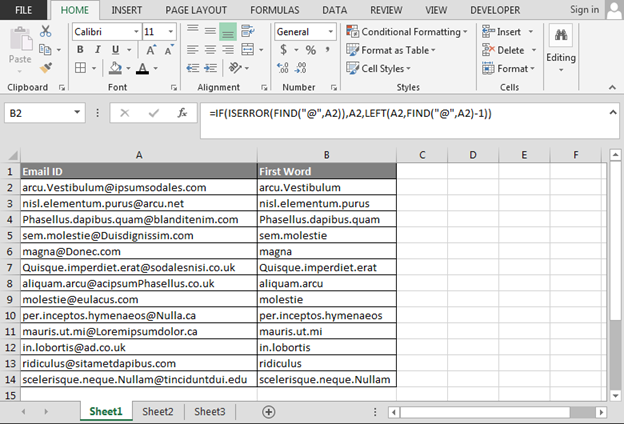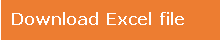# Excel formula to extract the first word in a cell

In this article, we are going to learn Excel formula which we can use to get the first word from a cell.

To resolve this query, we will use IF, ISERROR, FIND and LEFT functions.

In this situation, IF function will help to check the multiple conditions at one time and will help to get the result.

ISERROR function will help to give the situation if result is any error.

FIND function will help to find the symbol after first word.

LEFT function will pick the value from the left side.

Let’s take an example to understand.

We have email id’s data in Excel, and we want to retrieve the first word or name from the email id’s.• Enter the formula in cell B2.
• =IF(ISERROR(FIND("@",A2)),A2,LEFT(A2,FIND("@",A2)-1))
• Press Enter.
• Copy formula in the range.• First Name or first word will be extracted from the column.

Note:- If you want to extract first word before the space, then you just replace @ with space in the formula, and then you can apply.

This is the way we can make the customize formula according to our data and requirement in Microsoft Excel.1.